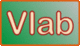online_pump_pipe_system:  Operating discharge and head in a pump-pipe systemDefinition sketch of a pump-pipe system

 SAMPLE INPUT     SI units [U.S. Customary units] Pipe diameter D (m) [ft]:  0.5 [1.6404] Pipe length L (m) [ft]:  2000 [6,561.7 ft] Friction factor f =  0.016 Upstream reservoir elevation E1 (m) [ft]:  450 [1,476.4] Downstream reservoir elevation E2 (m) [ft]:  500 [1,640.4] Entrance loss coefficient Ke =  0.50 Exit loss coefficient KE =  1.00 Sum of bend loss coefficient(s) Kb =  0.19 (one bend, r/d = 2) Type 1 head-discharge relation:  (Theoretical H-Q head-discharge relation, or pump curve) c (m) [ft] :  70 [229.66] d (s2/m5) [s2/ft5] :  700 [1.8415] Type 2 head-discharge relation:  (Practical H-Q head-discharge array) N = 8 H (i) (m) [ft] :  70, 60, 50, 40, 30, 20, 10, 0  [229.659,196.850,164.042,131.234,98.425,65.617,32.808,0.] Q (i) (m3/s) [cfs] :  0., 0.11952, 0.16903, 0.20702, 0.23905, 0.26726, 0.29277, 0.31623  [0.,4.2208,5.9692,7.3108,8.4420,9.4382,10.3391,11.1676] OUTPUT Type 1 head-discharge relation: •  Operating discharge Q (m3/s) [cfs] :  0.1594 [5.6319] •  Operating head H (m) [ft] :  52.2074 [171.2503] Type 2 head-discharge relation: •  Operating discharge Q (m3/s) [cfs] :  0.1583 [5.5907] •  Operating head H (m) [ft] :  52.1751 [171.1445] The minor differences between the two methods are due to the interpolating accuracy in the Type 2 head-discharge relation.

Thank you for running online_pump_pipe_system.